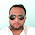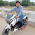## Saturday, December 27, 2014

### Measurements and Instrumentation Objective Questions with Answers: Part-9

MEASUREMENTS AND INSTRUMENTATION OBJECTIVE QUESTIONS WITH ANSWERS
 A rectifier type instrument is connected to 100VDC and is operated in the DC measuring module reads
(a) 111V
(b) 90V
(c) 50V
(d) 100V

 A permanent magnet moving coil ammeter has a coil resistance of 99ohm and Full Scale Deflection(FSD) current of 0.1mA. Shunt resistance is 1 ohm. Current through the meter at 0.5 F.S.D is
(a) 0.007mA
(b) 0.05mA
(c) 0.023mA
(d) 0.1mA

 One single phase wattmeter operating on 230V and 5A for 5 hours makes 1940 revolutions. Meter constant in revolutions is 400. What is the power factor of the land?
(a) 1
(b) 0.84
(c) 0.73
(d) 0.65

 For power measurement of three phase circuit by two wattmeter method, when the value of power factor is less than 0.5 lagging
(a) one of the wattmeters will read zero
(b) both give the same readings
(c) one of the wattmeter connections will have to be reversed
(d) pressure coil of the wattmeter will become ineffective.

 When using ohmmeter, applied voltage is to be disconnected from the circuit because
(a) Voltage source will increase resistance
(b) Current will decrease resistance
(c) the ohmmeter has its own internal battery
(d) non of the above

 Which wave has the least form factor?
(a) Square wave
(b) Rectangular wave
(c) Sine wave
(d) Triangular wave

 With a sweep time 10ms across the screen the approx. horizontal sawtooth frequency will be
(a) 50Hz
(b) 100Hz
(c) 1kHz
(d) 500Hz

 A spring controlled indicating instrument has a deflection of 90deg for maximum current I. The corresponding deflection for a gravity controlled instrument is
(a) 90°
(b) 120°
(c) 30°
(d) 0°

 It is found that 10A current passes through a resistor of 10ohms. The resistor has 5% tolerance . The error in measurement of current can be as high as 5%. What is max error in measurement of power, calculated from measured value of I and the nominal value of resistor?
(a) 100 W
(b) 150W
(c)50W
(d) 200W

 A resistor of 10Kohms with 5% tolerance is connected in parallel with a 5Kohms resistor of 10% tolerance. What is the tolerance limit for the parallel network?
(a) 5%
(b) 6.67%
(c) 10%
(d) 8.33%

 The resistance offered to the passage of an in-varying current by a column of mercury at the temperature of melting ice of mass 14.4521 gms of uniform cross-sectional area and length 106.3cm is
(a) Standard ohm
(b) One international ohm
(c) One ohm according to SI units
(d) One ohm according to working standards
12:52 AM

#### 3 comments:

1.2.Please tell me how u solved this problems...atlst Tel me the formulas

3.